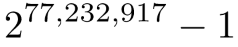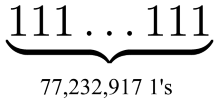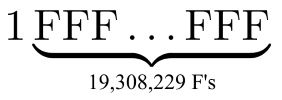# New prime number record: 50th Mersenne prime

A new record for the largest known prime was announced yesterday:This number has 23,249,425 digits when written in base 10.

(Update: A new record was announced December 21, 2018. More on it here.)

In base 2, 2p – 1 is a sequence of p ones. For example, 31 = 25 -1  which is 11111 in binary. So in binary, the new record prime is a string of 77,232,917 ones.You can convert a binary number to hexadecimal (base 16) by starting at the right end and converting blocks of 4 bits into hexadecimal. For example, to convert 101101111 to hex, we break it into three blocks: 1, 0110, and 1111. These blocks convert to 1, 6, and F, and so 101101111 in binary corresponds to 16F in hex.

Now 77,232,917  = 19,308,229 * 4 + 1, so if we break our string of 77,232,917 ones into groups of four, we have a single bit followed by 19,308,229 groups of 4. This means that in hexadecimal, the new prime record is 1FFF…FFF, a 1 followed by 19,308,229 F’s.The new record is the 50th Mersenne prime. A Mersenne prime is a prime number that is one less than a power of 2, i.e. of the form 2p – 1. It turns out p must be prime before 2p – 1 can be prime. In the case of the new record, 77,232,917 is prime.

It is unknown whether there are infinitely many Mersenne primes. But now we know there are at least 50 of them.

All the recent prime number records have been Mersenne primes because there is an algorithm for testing whether a number of the special form 2p – 1 is prime, the Lucas-Lehmer test.

Mersenne primes are closely related to perfect numbers. A number n is perfect if the sum of the numbers less than n that divide n equals n. For example, 28 is divisible by 1, 2, 4, 7, and 14, and 1 + 2 + 4 + 7 + 14 = 28.

Finding a new Mersenne prime means finding a new perfect number. If m is a Mersenne prime, then nm(m + 1)/2 is a perfect number. Conversely, if n is an even perfect number, n has the form m(m + 1)/2, Nobody knows whether odd perfect numbers exist. Since we don’t know whether there are infinitely many Mersenne primes, we don’t know whether there are infinitely many perfect numbers. But there are at least 50 of them.

## One thought on “New prime number record: 50th Mersenne prime”

1. Hollie

At this point, I don’t believe it’s been verified that there are no smaller Mersenne primes. So it’s the 50th one known, but not known to be the 50th.